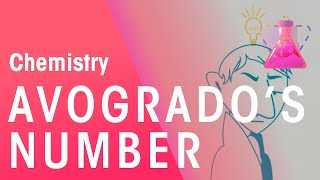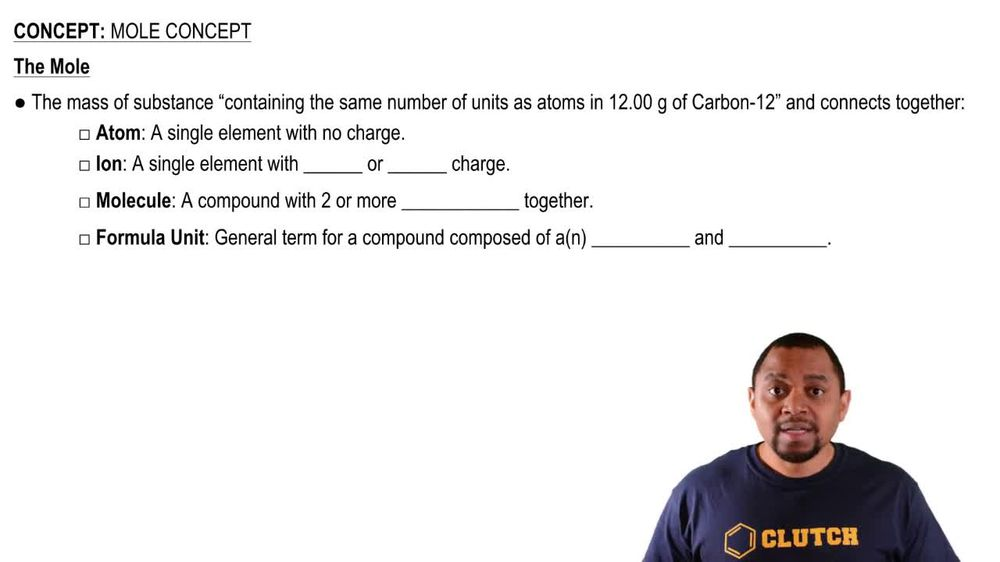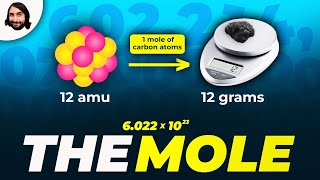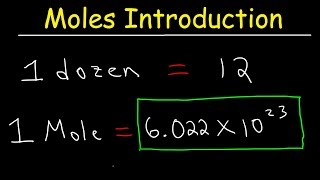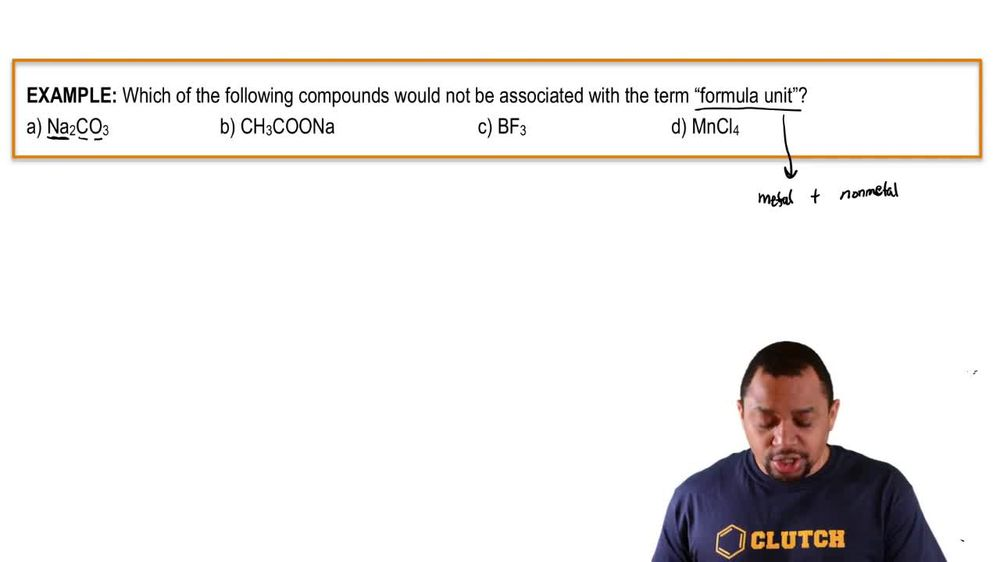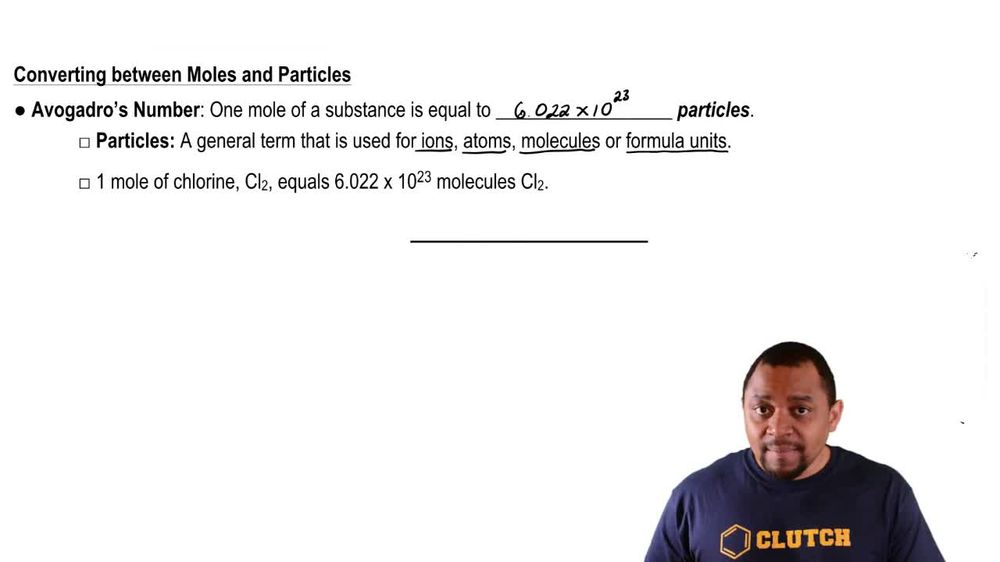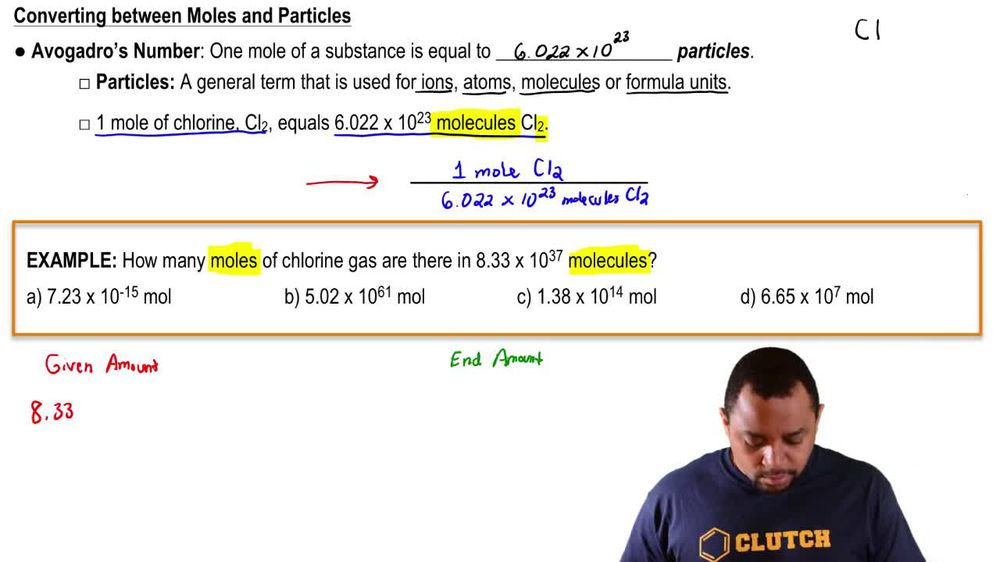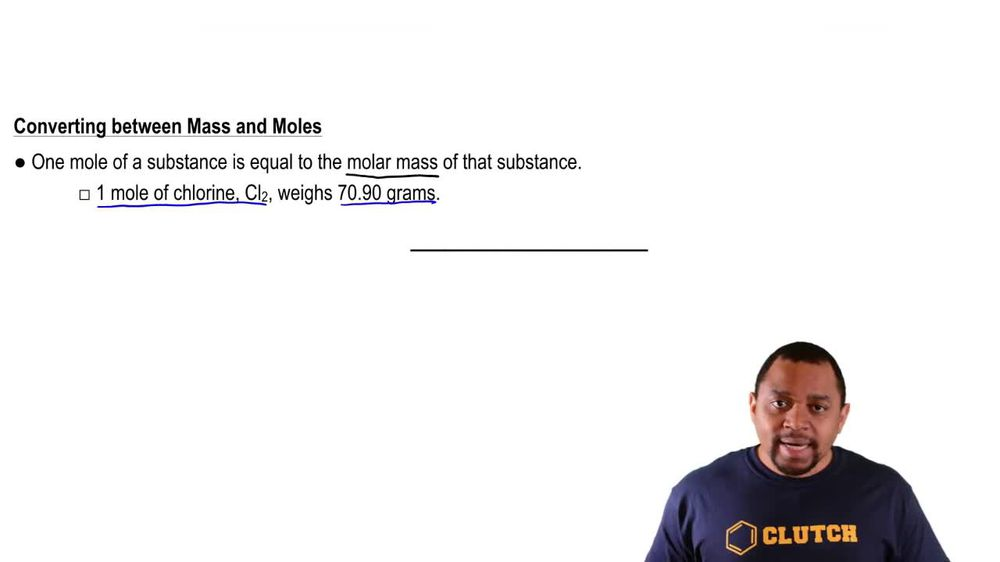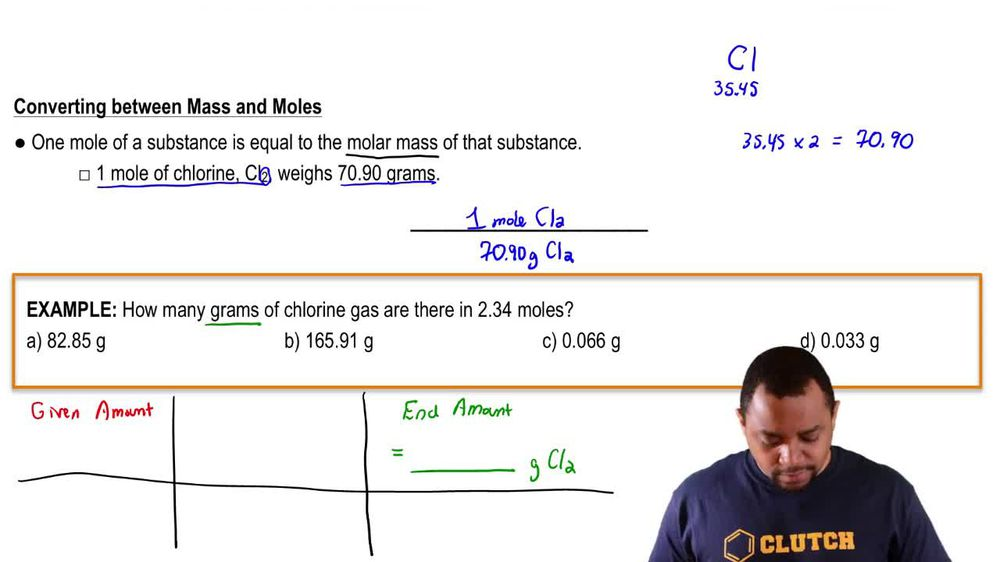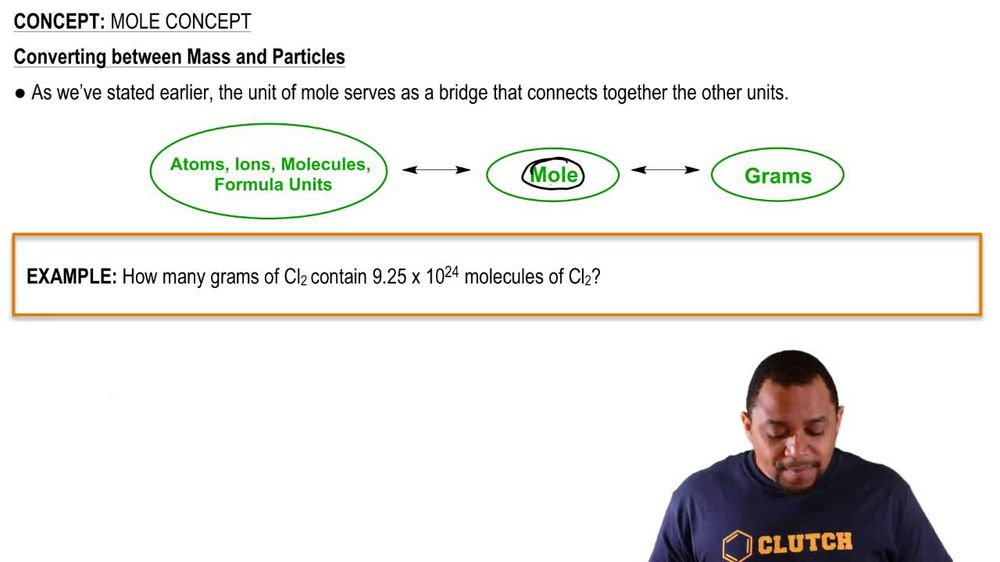Start typing, then use the up and down arrows to select an option from the list.
1. 2. Atoms & Elements2. Mole Concept
Problem

# (a) What is the mass, in grams, of 1.223 mol of iron(III) sulfate? (b) How many moles of ammonium ions are in 6.955 g of ammonium carbonate? (c) What is the mass, in grams, of 1.50 * 1021 molecules of aspirin, C9H8O4?

Relevant Solution7m
Play a video:
Hi everyone here we have a question asking us to first calculate the mass and grams of 2.639 moles of cobalt carbonate. To calculate the moles of ammonium ions in 9. g of ammonium sulfate and three calculate the mass and grams of 8.69 times 10 to the 22nd mole molecules of caffeine C eight H 10 N 402. So we're gonna start off here with one and we have cobalt which has a charge of plus three and carbonate which has a charge of minus two. And we're going to crisscross these to give us cobalt two carbon 83. And now we need to add the molecular mass of that. So we have cobalt two times the molecular mass found on the periodic table, 58.93 equals 117. Carbon three times 12.01 equals 36.03, oxygen nine times equals 144. And when we add that all up, we get 297.89 g per mole. And now we can calculate the mass in grams. So we're going to start off with our two . moles of cobalt carbonite. And we're going to multiply by .94g. And that's Permal So our moles are canceling out and that equals 786. g of cobalt carbonate. So that is our answer for one. and now moving on to two, we have ammonium sulfate, which is N H four two S. 04. And we need to calculate its molecular mass. So we have nitrogen Which is 14.01 and we have two of them, so times two That equals 28.02. We have hydrogen which is 1.01 times eight which is 8.08. We have sulfur which is 32.07, and we have oxygen which is 16 times four which equals 64. When we add all of these, we get 132.17 g per mole. So we have nine .63, 1 g of ammonium sulfate times one mold of ammonium sulfate over the molar mass 132.17 grams of ammonium sulfate, times two moles of ammonium over one mole of ammonium sulfate. And that's because we have two modes of ammonium for every one mole of ammonium sulfate and that equals 0.1457 moles of ammonium. And that is our answer for part two. Lastly we have part three and we need to calculate the mass of 8.69 times 10 to the 22nd molecules of caffeine. So we first need to add up the molar mass. So we have carbon 12. Times eight equals 96.08. We have hydrogen 1.01 times 10 equals 10.1. We have nitrogen 14. times four equals 56.4 We have oxygen 16 times two equals For a total of 194. g per mole. And we're going to take 8. Times 10 to the 22nd molecules, A caffeine Times one mole of caffeine over Avocados No. So 6.02, 2 times 10 to the 23rd molecules And then times 194 points to two g Permal. And our molecules are canceling out. Our moles are canceling out, giving us 28. grams of caffeine. And that is our third and final answer. Thank you for watching. Bye.